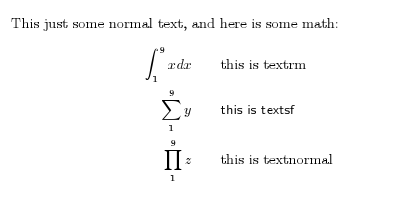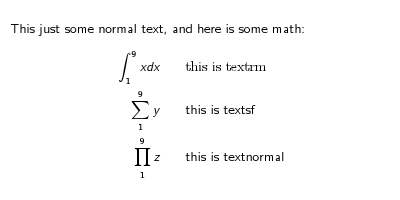If you you want to use normal text in a math environment (mathdisplay, equation,...) you should use \textnormal{} instead of \textrm{}. Apparently the latter is more popular: a google search for "latex textrm" delivers almost 25.000 hits, whereas google search for "latex textnormal" returns not more than 800 hits. The advantage of \textnormal is that the text will be typeset in the normal font of the body text. \textrm on the other hand activates a roman (serif) font. Most of the time the result of these two will be the same because the default font of the body text is a serif one in most cases. But sometimes it is not.

An example I encountered was reusing LaTeX code from an article in a LaTeX Beamer Class presentation. In a beamer presentation the default font is a sans serif one. When I reused my math expressions, I had to replace all \textrm with \textsf to adjust to this "other default". If I would have used \textnormal, there wouldn't be a problem.

For example (I like examples), consider the following LaTeX body, saved to a file textnormalexample_body.tex:

This just some normal text, and here is some math:
\begin{eqnarray*}
\int_1^9 x dx & & \textrm{this is textrm}\\
\sum_1^9 y    & & \textsf{this is textsf}\\
\prod_1^9 z   & & \textnormal{this is textnormal}
\end{eqnarray*}


We use this in a simple article document:

\documentclass{article}
\begin{document}
\input {textnormalexample_body.tex}
\end{document}


and a comparable simple beamer presentation:

\documentclass{beamer}
\begin{document}
\begin{frame}
\input{textnormalexample_body.tex}
\end{frame}
\end{document}


The result of the article is:The result of the beamer presentation is:Note that the font of the text inside the \textnormal{..} matches the font of the body in both cases.